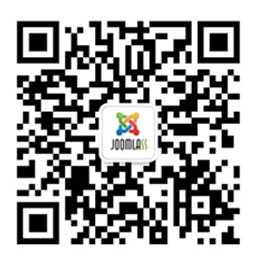# 在粒子中使用CMS

### 所有人都有共同的功能

#### 创建一个新的查询

``````{% set options = {published: true, id: [14, 3]} %}
{% set finder = joomla.finder('category', options) %}``````
##### .parse(options)解析选项数组到规则

``````{% set options = {published: true, id: [14, 3]} %}
{% do finder.parse(options) %}``````
##### .find()使用分页查找项

``{% set results = finder.find() %}``
##### .count()计算项目总数

``Total count of items: {{ finder.count() }}``

### 操作

##### .start(limitstart = 0)分页开始

``{% do finder.start(10) %}``
##### .limit(limit = 10)分页限制

``{% do finder.limit(3) %}``
##### .order(by, direction = 'ASC', alias = 'a')页码顺序

``{% do finder.order('id', 'DESC') %}``
##### .where(field, operation, value)定制的where子句

``````{% do finder.where('id', '<', 5) %}
{% do finder.where('id', 'BETWEEN', [1,5]) %}
{% do finder.where('id', 'NOT IN', [1,2,3,4,5]) %}``````

### 分类查找

#### 创建一个新的类别查找器

``````{% set options = {published: true, id: [[14,15], 3]} %}
{% set finder = joomla.finder('category', options) %}``````
##### .id(ids, include = true)
• `ids` 要么是id数组，要么是单个id。
• `include` True如果你想要包括列出的类别。false 如果你想把它们排除在外
``{% do finder.id([1,2,3]) %}``
##### .language(language = true)

language如果使用用户默认值，则使用语言标记。

``{% do finder.language('en-GB') %}``

### 内容搜索

#### 创建一个新的内容查找器

``````{% set options = {featured: true, id: [[1,2,3]]} %}
{% set finder = joomla.finder('content', options) %}``````
##### .id(ids, include = true)
• `ids` 要么是id数组，要么是单个id
• `include` True 如果您想要包含列出的文章，false如果你想把它们排除在外
``{% do finder.id([1,2,3]) %}``
##### .featured(include = true)

include如果你想要包含有特色的文章，如果你想要把它们排除在外，设置为真

``{% do finder.featured() %}``
##### .author(ids, include = true)
• `ids` 要么是id数组，要么是单个id
• `include` True 如果您想要包含列出的文章，false如果你想把它们排除在外
``{% do finder.author(64) %}``
##### .language(language = true)

language如果使用用户默认值，则使用语言标记。

``{% do finder.language('en-GB') %}``

##### .category(ids, include = true)
• `ids` 要么是id数组，要么是单个id
• `include` True 如果您想要包含列出的文章，false如果你想把它们排除在外
``{% do finder.category([1,2,3]) %}``

### 类对象

#### 额外的字段

##### .route分类路径

``<a href="{{ category.route }}">{{ category.title }}</a>``

#### 操作

##### .parent()获得父类对象

``{% set parent = category.parent() %}``
##### .parents()获取所有父类对象

``{% set parents= category.parents() %}``
##### .compile(string)将类别编译成字符串

``{{ category.render('<a href="/{{ category.route }}">{{ category.title }}</a>') }}``
##### .render(template)呈现类别

``{{ category.render('joomla/category-link.html.twig') }}``

``<a href="{{ category.route }}">{{ category.title }}</a>``

### 内容对象

#### 额外的字段

##### .text 引言+全文

``{{ article.text|raw }}``

``{{ article.readmore ? 'Read More...' }}``
##### .route 文章路径

``<a href="{{ article.route }}">{{ article.title }}</a>``

#### 操作

##### .author()获得作者对象

``{% set author = article.author() %}``
##### .category()获取类对象

``{% set category = article.category() %}``
##### .categories()得到所有类别对象

``{% set categories = article.categories() %}``
##### .compile(string)将文章编译成字符串

``{{ article.render('<a href="/{{ article.route }}">{{ article.title }}</a>') }}``

.render(template)呈现文章 使用给定的模板文件呈现文章。模板context有一个名为article的变量，您可以使用它。

``{{ article.render('joomla/article-link.html.twig') }}``

``<a href="{{ article.route) }}">{{ article.title }}</a>``

#### 访问当前文章

``````{% set request = gantry.request.request %}
{% if request['option'] == 'com_content' and request['view'] == 'article' and request['id'] %}

{% set article = joomla.finder('content').id([request['id']]).language().find()|first %}
<h1>{{ article.title }}</h1>

{% endif %}``````

• #### 主题

• QQ在线客服点击按钮咨询
• 服务热线010-8646 9696
工作时间：早9:00 - 晚18:00
•微信在线客服